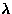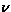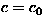Next: 5.3 Summary Up: 5.2 Haldane material YBaNiO Previous: Unconventional dynamics in the

### Paramagnetic fluctuations in the pure and Mg doped systems

From the longitudinal field (LF) dependence of the muon spin relaxation rate (), it was shown that the nominally pure and the vacancy doped systems stay paramagnetic down to the milli-Kelvin regime (Fig.42). The doping dependence of the Lorentzian width (a) and the field fluctuation rate ()qualitatively suggests that muons are detecting the dipolar fields from the unpaired spins (see Table 5). In this section, a more quantitative discussion is given.

In order to estimate the numbers of unpaired spins, we analyzed the paramagnetic region of the susceptibilities with the Curie-Weiss law, a model which assumes that all the doping effect is a creation of local moments. The results are shown in Fig.47.In the vacancy doped systems, the Mg concentration (y) dependence of the Curie constant (C) is consistent with the heuristic `singlet-triplet' model, which assumes that two Mg2+ ions effectively create one S=1 spin . In the charge doped systems, one Ca ion seems to create=1 spin, or=1/2 spins. Since susceptibility has no information about the local structure of the unpaired spins, it is not possible to distinguish these two situations. For simplicity, we assume hereafter that=1 effective spins are created for one Ca ion, and=1 spins for one Mg ion.

It is known that the paramagnetic moments of the nominally pure Y2BaNiO5 are created by excess oxygen, which works as a hole-dopant . The Curie constant of the nominally pure specimen corresponds to the native charge concentration ofat.%, which has been estimated by extending the Ca concentration dependence of the Curie constant (Fig.47a) to the negative x side.

In order to estimated the Lorentzian field width (a) generated from the unpaired spins, we utilized the procedure developed for analysis of the dilute spin-glass alloys :

(1)
obtain the hypothetical Gaussian field width () for the situation in which all the spin sites are filled up with randomly oriented moments. In zero external field, this Gaussian width () is expressed as :
where, S is the size of the spins at each site,() is the muon(electron) gyromagnetic ratio. (, where g is the g-factor andis the electron Bohr magneton.)
(2)
using eq.26, obtain the Lorentzian width (a) from the hypothetical Gaussian width.

Since we do not know the muon site in Y2BaNiO5, we assumed locations shown in Fig.48. These sites assumed are allÅ away from a oxygen ion, where a muon usually resides in oxides [113,114,115,116,117]. We numerically performed the dipolar sum (eq.45), assuming that the g-factor is 2 for the doping induced S=1 spins. The results () are shown in Fig.48.If an external field is applied to a paramagnetic spin system, the electron moments undergo Larmor precession. Since the gyromagnetic ratio of muon and electron differ by two orders of magnitude, the precessing electron spin component becomes invisible to a muon. Namely, only the secular part of the dipolar field contributes to muon spin relaxation in longitudinal field measurements. For paramagnetic T1 relaxation, the secular contribution is 3/10 of the zero-field T1 relaxation rate (the 10/3-effect [9,10]; see section A.2). Therefore, the hypothetical Gaussian width appropriate to the longitudinal field measurements () becomes:

Using the Gaussian width, the estimated unpaired spin concentration(y is the Mg concentration) and eq.26, the Lorentzian field widths (a) were estimated as shown in Table 5. Although the ambiguity from the muon site is large, the experimental data is within the range of the estimated magnitude.

If the fluctuations of the unpaired spins are governed by their dipolar interactions, the fluctuation rate () should scale with the Lorentzian width (a). The ratiowill be the order of the ratio of the electron/muon gyromagnetic factors (), where the factorcomes from the fact that an electron moment detects the entire spin of other electrons, and the factorcorrects the units (a in rad/sec andin Hz). The fluctuation rates estimated as above are shown in Table 5. The experimental results again are in the same order of magnitudes with these estimates.

The above estimates of the field width (a) and the fluctuation rate () in the nominally pure and Mg-doped systems supports the scenario in which the muon detects the dipolar fields of the unpaired spins in these systems.Next: 5.3 Summary Up: 5.2 Haldane material YBaNiO Previous: Unconventional dynamics in the